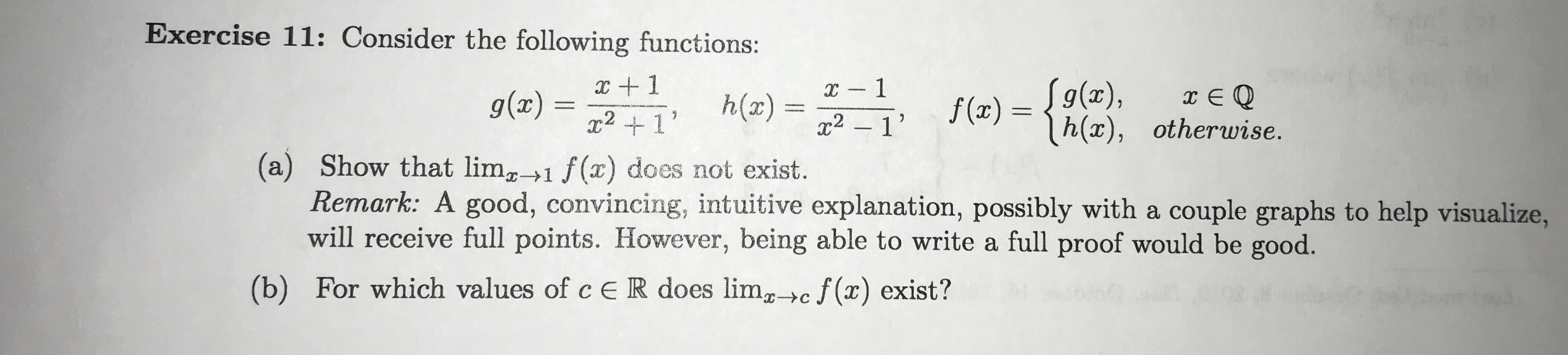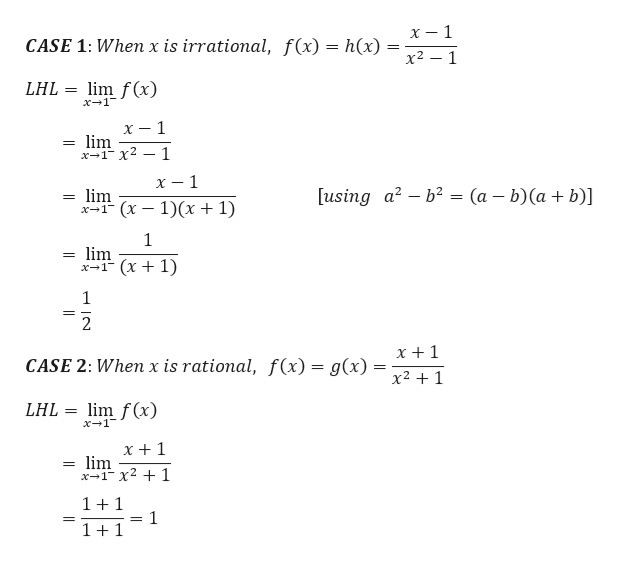# Exercise 11: Consider the following functions:x -12 11S9(x),h(x), otherwise.g(x)h(x) =f (x)x21(a) Show that limg1 f(x) does not exist.Remark: A good, convincing, intuitive explanation, possibly with a couple graphs to help visualize,will receive full points. However, being able to write a full proof would be good.(b) For which values of c ER does lim>c f(x) exist?

Questionhelp_outlineImage TranscriptioncloseExercise 11: Consider the following functions: x -1 2 1 1 S9(x), h(x), otherwise. g(x) h(x) = f (x) x21 (a) Show that limg1 f(x) does not exist. Remark: A good, convincing, intuitive explanation, possibly with a couple graphs to help visualize, will receive full points. However, being able to write a full proof would be good. (b) For which values of c ER does lim>c f(x) exist? fullscreen
check_circle

Step 1

NOTE – As you have uploaded multiple sub-parts under a single question, we will answer the first sub-part for you. Reupload other sub-parts separately in case you need help with their solution.

We need to find the limit of the f(x) at x = 1, where f(x) is

Step 2

In the neighbourhood of x = 1, we can encounter infinite numbers of rational and irrational numbers. Thus, the...help_outlineImage Transcriptioncloseх — 1 CASE 1: Whenx is irrational, f(x) = h(x) х2 — 1 LHL= lim f(x) x1 х — 1 lim х-1- х2 — 1 х — 1 using a2-b2 = (a - b)(a + b)] lim -т (х — 1)(х + 1) 1 lim х-1 (х + 1) 1 2 x +1 CASE 2: When x is rational, f(x) = g(x) x21 LHL = lim f(x) x 1 x +1 lim x1 x2 1 = 11 1 1+1 = N fullscreen

### Want to see the full answer?

See Solution

#### Want to see this answer and more?

Solutions are written by subject experts who are available 24/7. Questions are typically answered within 1 hour.*

See Solution
*Response times may vary by subject and question.
Tagged in

### Limits